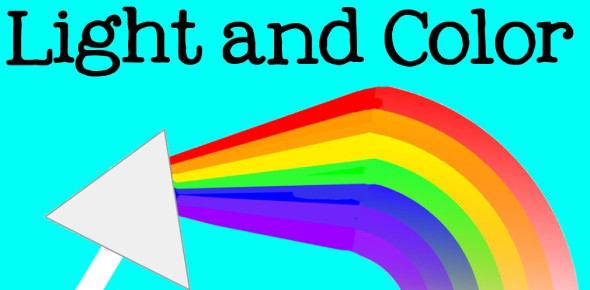# Light And Color

20 Questions | Total Attempts: 1799Settings.

• 1.
What color of light is produced when a primary color is combined with its complementary color?
• A.

Depends on the ratio of the combination

• B.

Black

• C.

Subdued version of the primary color

• D.

White

• 2.
Magenta is the complementary color to green. What two primary colors are used to form magenta?
• A.

red and green

• B.

red and blue

• C.

green and blue

• D.

Green and cyan

• 3.
Pigments rely on colors of light that are...
• A.

• B.

subtracted

• C.

Reflected.

• D.

Refracted.

• 4.
The primary pigments are _____ the primary colors.
• A.

Complementary to

• B.

Associated with

• C.

The inverse of

• D.

Unrelated to

• 5.
When red light and green light shine on the same place on a piece of white paper, the spot appears to be....
• A.

Yellow

• B.

Brown.

• C.

white.

• D.

black

• 6.
Which of the following is not an additive primary color?
• A.

Yellow

• B.

blue

• C.

Red

• D.

Green

• 7.
Which of the following is not a primary subtractive color?
• A.

Yellow

• B.

cyan

• C.

magenta

• D.

Blue

• 8.
When red light is compared with violet light,
• A.

both have the same frequency

• B.

both have the same wavelength

• C.

Both travel at the same speed.

• D.

red light travels faster than violet light.

• 9.
Which color combination is incorrect?
• A.

Blue + green = cyan

• B.

Blue + yellow = white

• C.

Red + yellow = magenta

• D.

Red + green = yellow

• 10.
Blue light, which is bent more than red light in a prism, has __________.
• A.

a shorter wavelength

• B.

A faster speed

• C.

a longer wavelength

• D.

A lower frequency

• 11.
What determines the color of light?
• A.

Wavelength

• B.

Frequency

• C.

Amplitude

• D.

Surface

• 12.
To make red light white, add __________.
• A.

Magenta light

• B.

Green light

• C.

Yellow light

• D.

Cyan light

• 13.
The mixing of primary colors of light to produce   other colors is a(n)__process.
• A.

Subtractive

• B.

Refractive

• C.

• D.

Diffractive

• 14.
Why can a prism break white light up into different colors?
• A.

Different colored light has different wavelengths

• B.

Certain parts of a prism only let certain colors of light pass through

• C.

The colors are changed by addition.

• D.

This is an example of color by subtraction

• 15.
Light behaves as __________.
• A.

A wave only

• B.

A particle and a wave

• C.

neither a particle nor a wave

• D.

A particle only

• 16.
A second prism will change a spectrum back into white light. This shows that __________.
• A.

Prisms distort images

• B.

White light is composed of colors

• C.

Prisms are transparent

• D.

Light travels at a constant speed

• 17.
Which of these properties of light is a constant?
• A.

Wavelength

• B.

frequency

• C.

Speed in a vacuum

• D.

Amplitude

• 18.
Choose the incorrect statement
• A.

Primary pigments reflects one color primary and absorbs two

• B.

Complementary colors are two colors that combine to form white

• C.

Primary colors combine to form other colors

• D.

A secondary color is a combination of two primary colors

• 19.
Bending of light around a barrier is called...
• A.

Refraction

• B.

Diffraction

• C.

Reflection

• D.

Polarization

• 20.
In what way do pigments act differently from light?
• A.

They produce different colors..

• B.

They reflect their color and absorb all the others.

• C.

They cannot be seen..

• D.

They produce only cyan, yellow, and magenta

Related Topics